# Selina Solutions Concise Maths Class 7 Chapter 4: Decimal Fractions (Decimals) Exercise 4C

Selina Solutions Concise Maths Class 7 Chapter 4 Decimal Fractions (Decimals) Exercise 4C provides students with the basic knowledge of multiplication and division of decimal numbers. Students can solve the problems on a regular basis and clear their doubts by referring to the solutions PDF. The solutions are strictly according to the current syllabus and marks weightage in the annual exam. Students can boost their confidence in solving tricky problems with the help of solutions PDF. Selina Solutions Concise Maths Class 7 Chapter 4 Decimal Fractions (Decimals) Exercise 4C, PDF links are provided here.

## Selina Solutions Concise Maths Class 7 Chapter 4: Decimal Fractions (Decimals) Exercise 4C Download PDF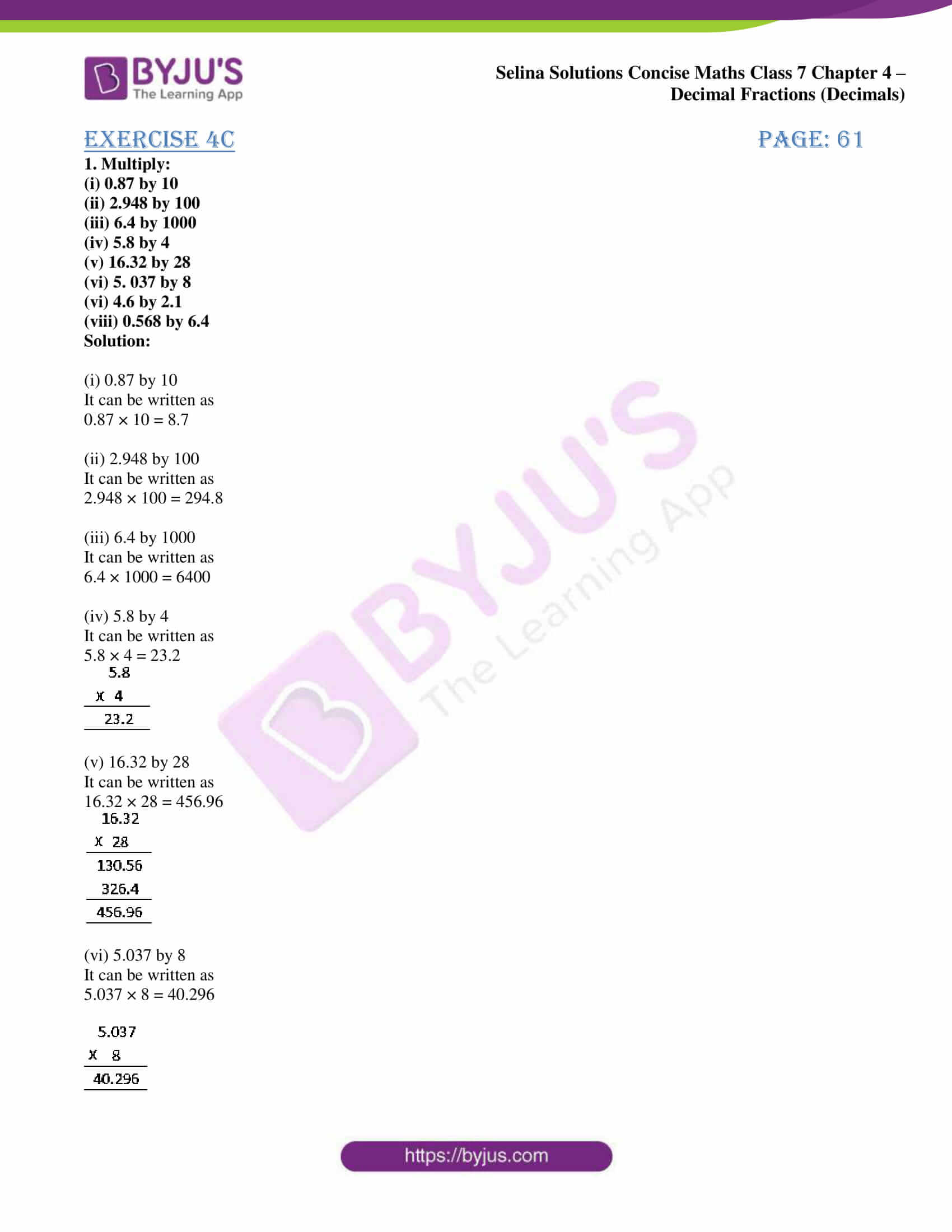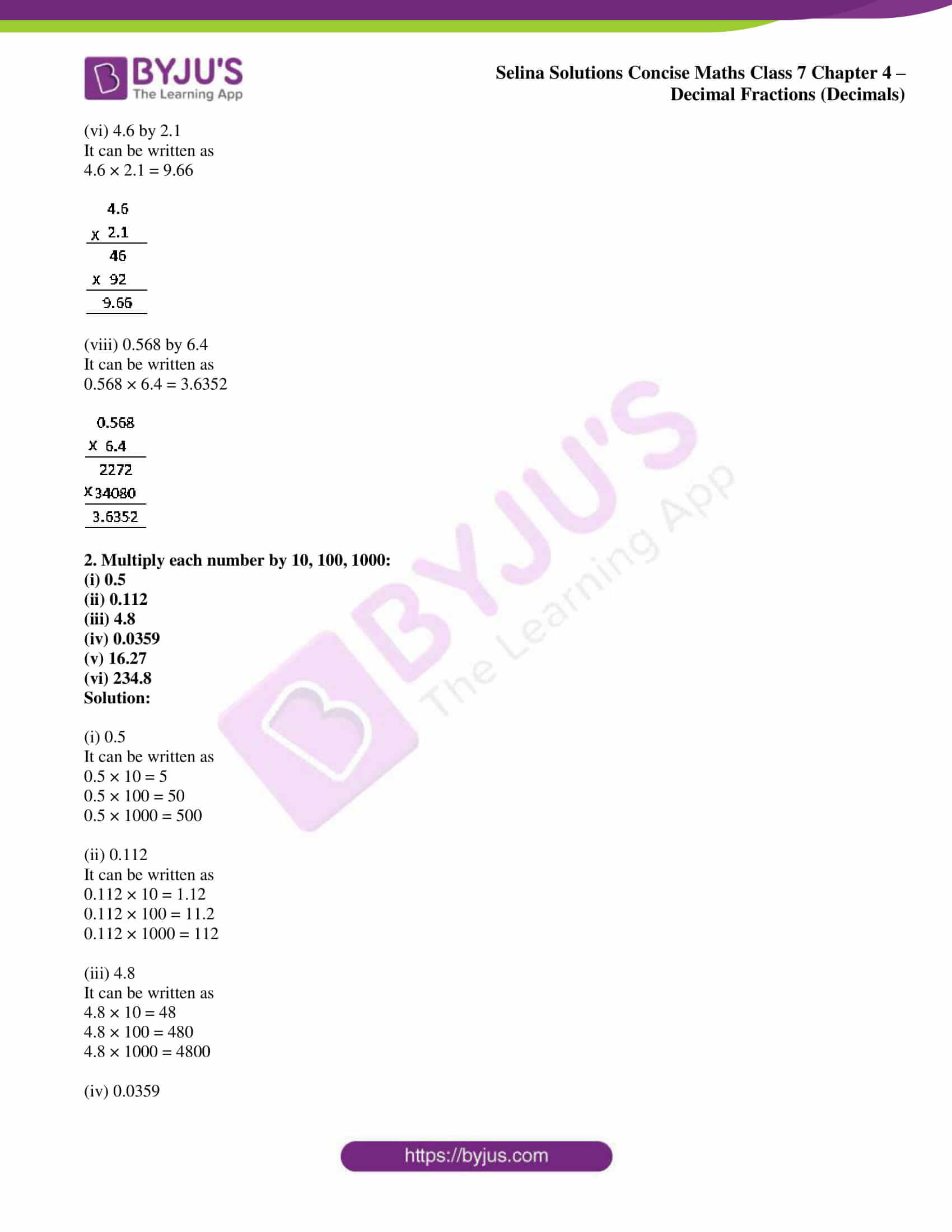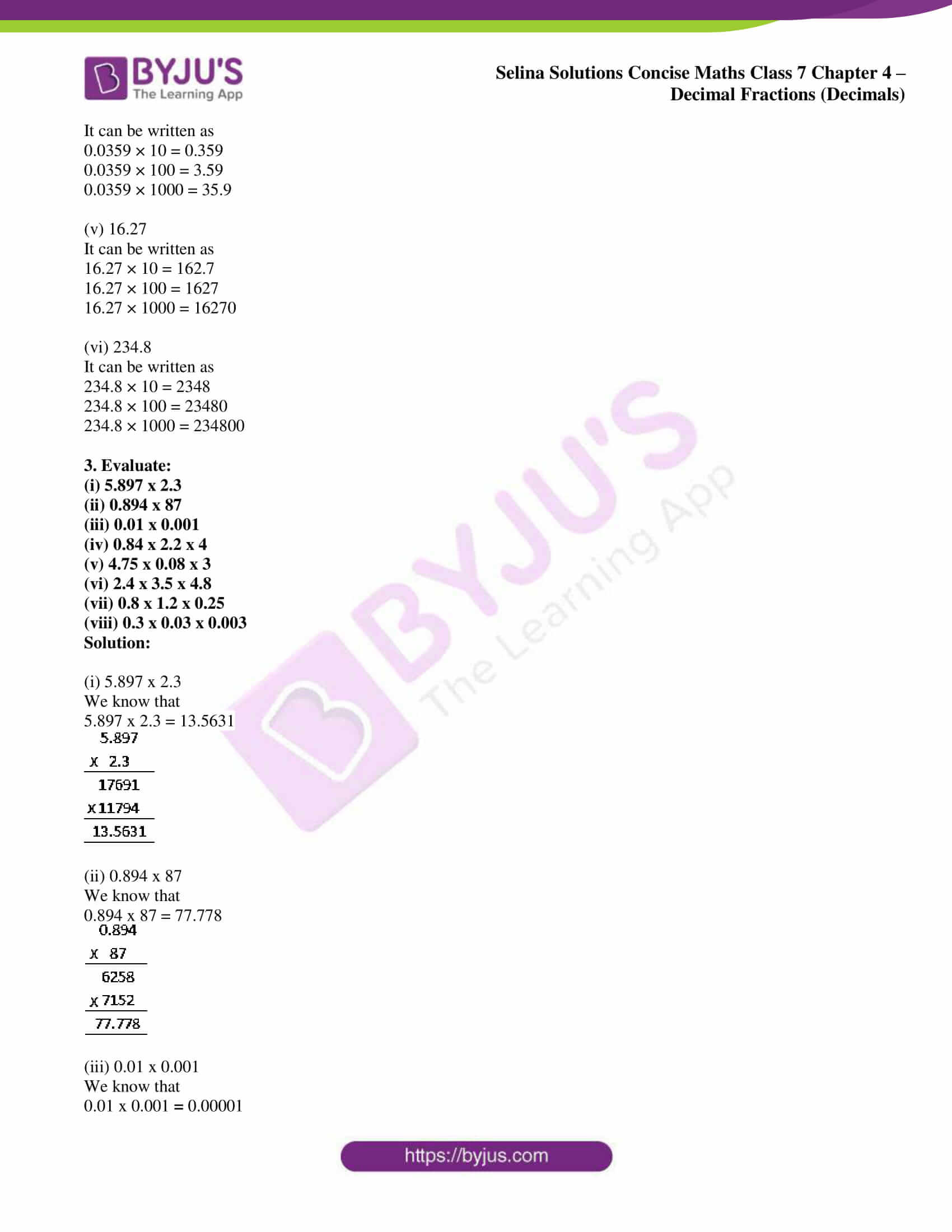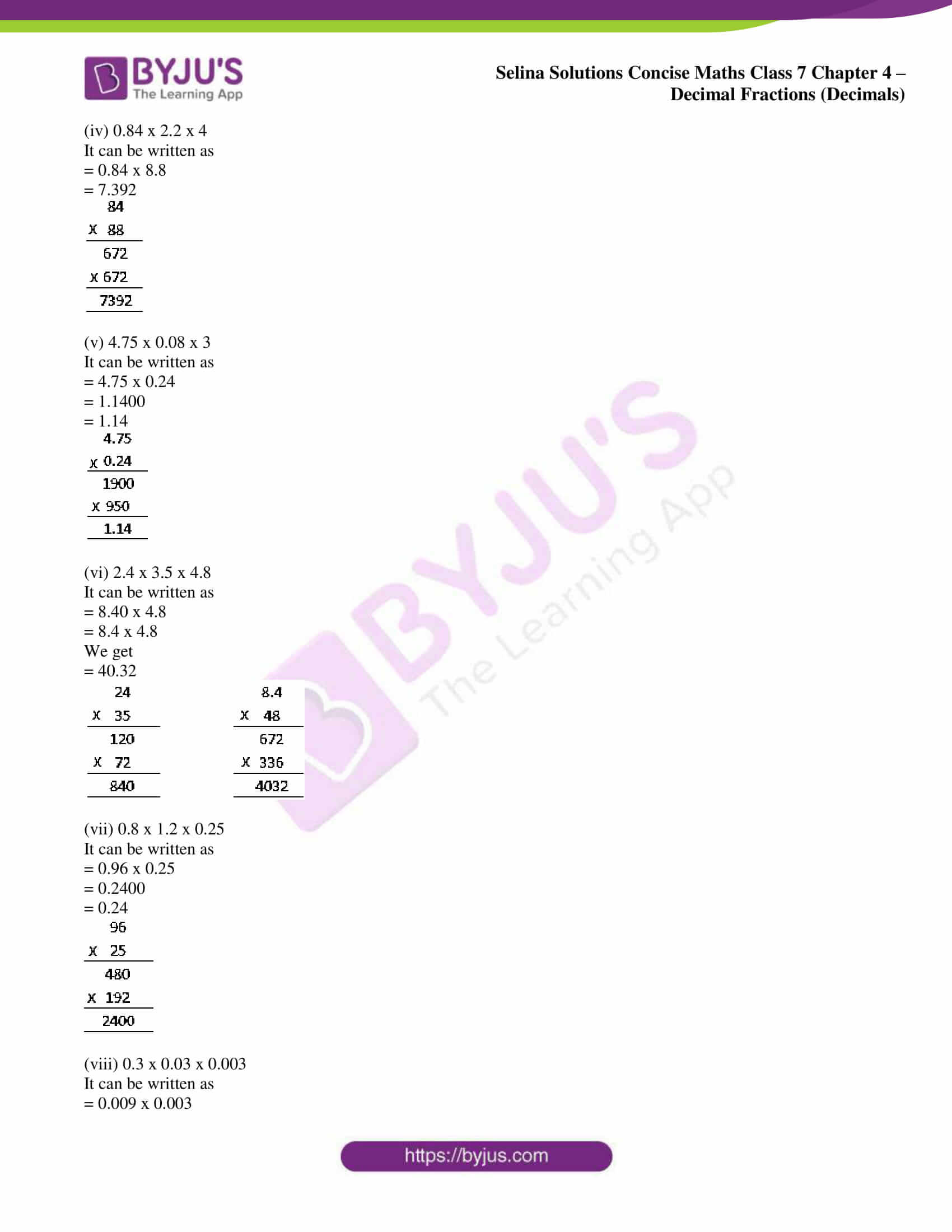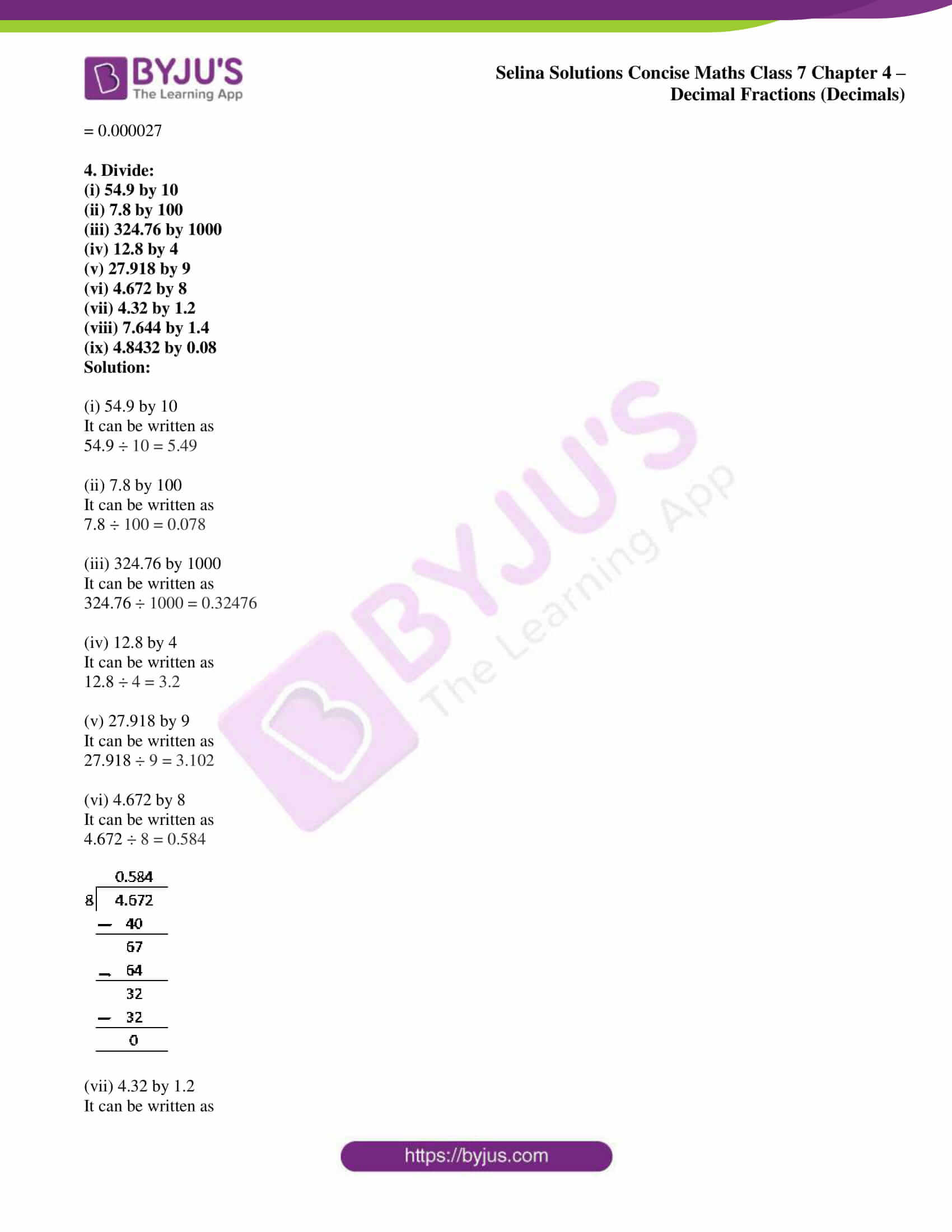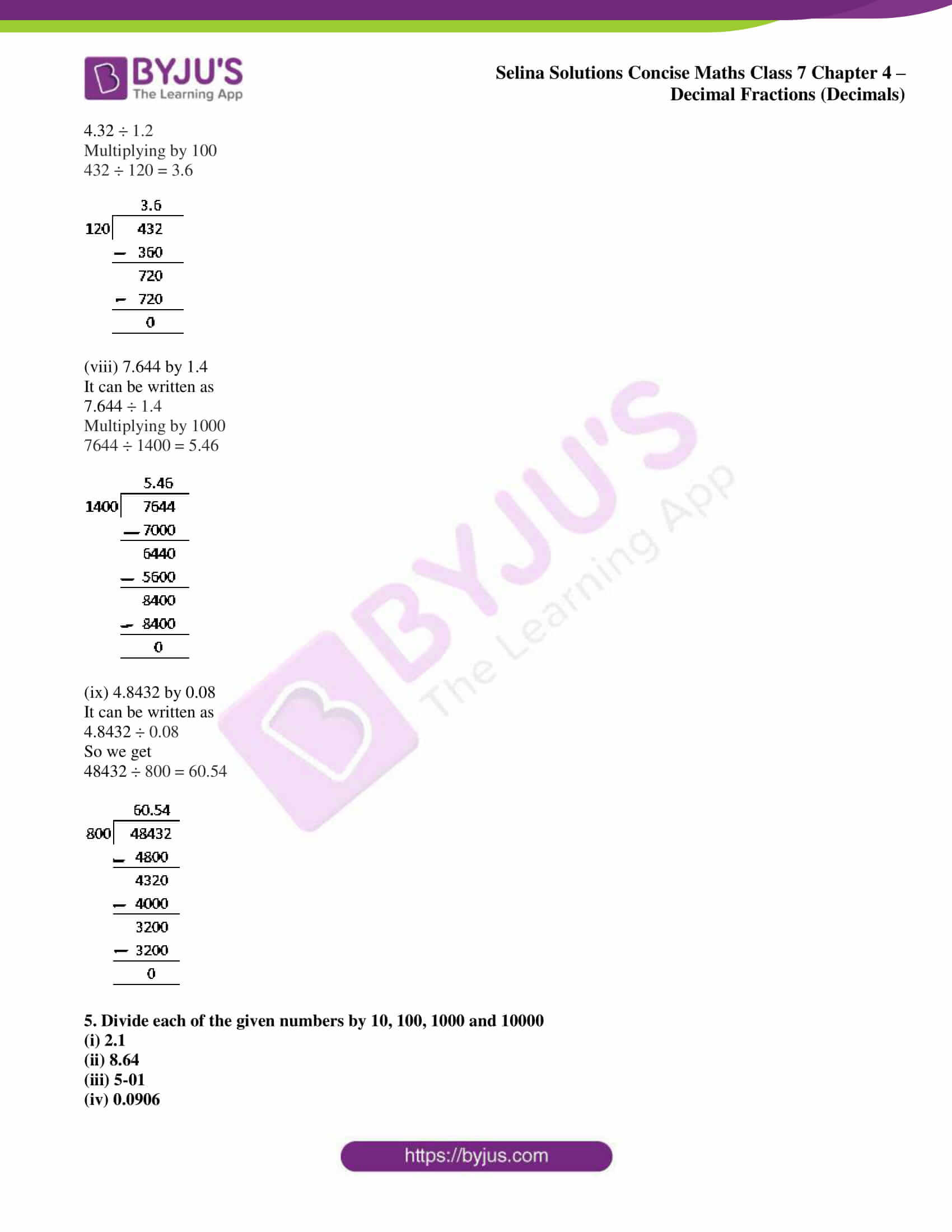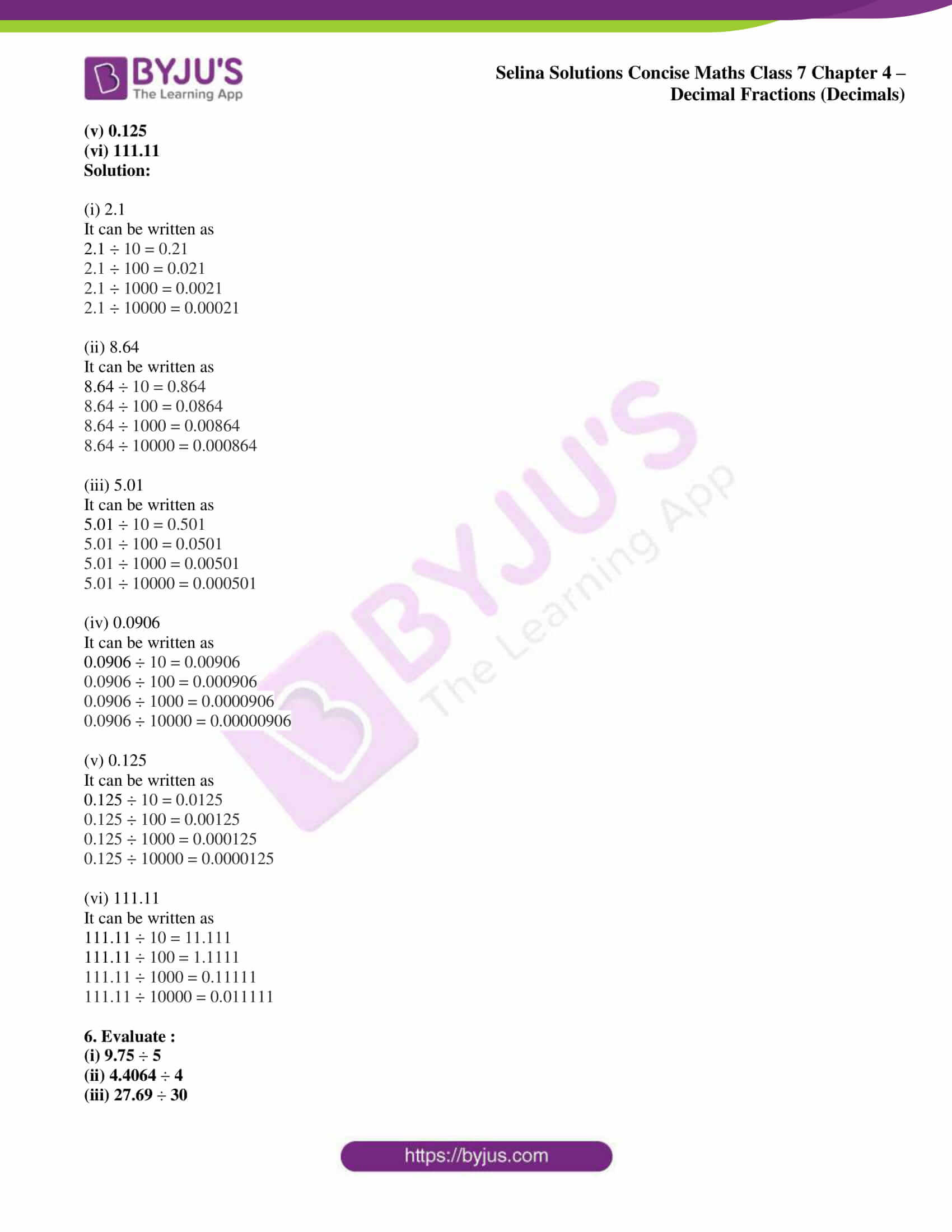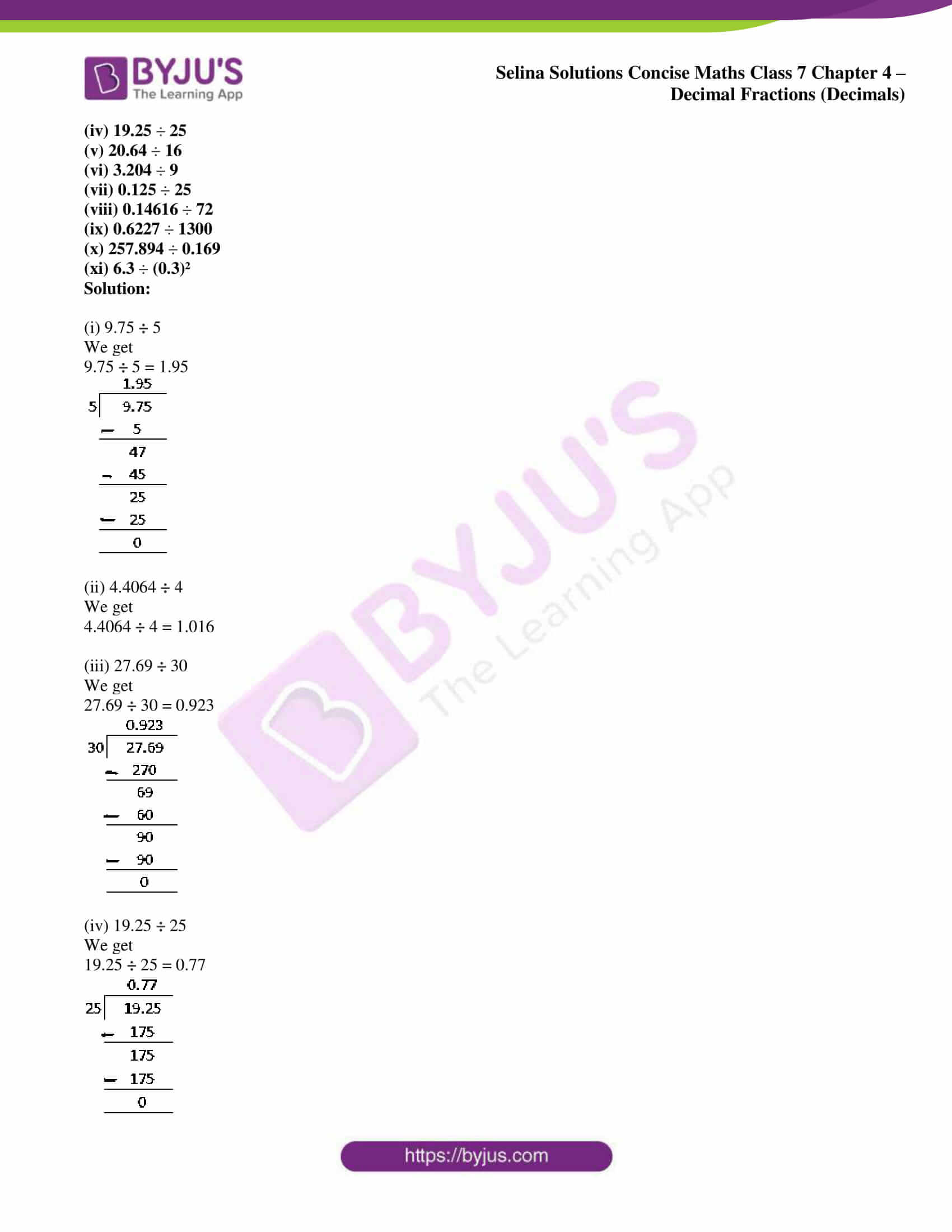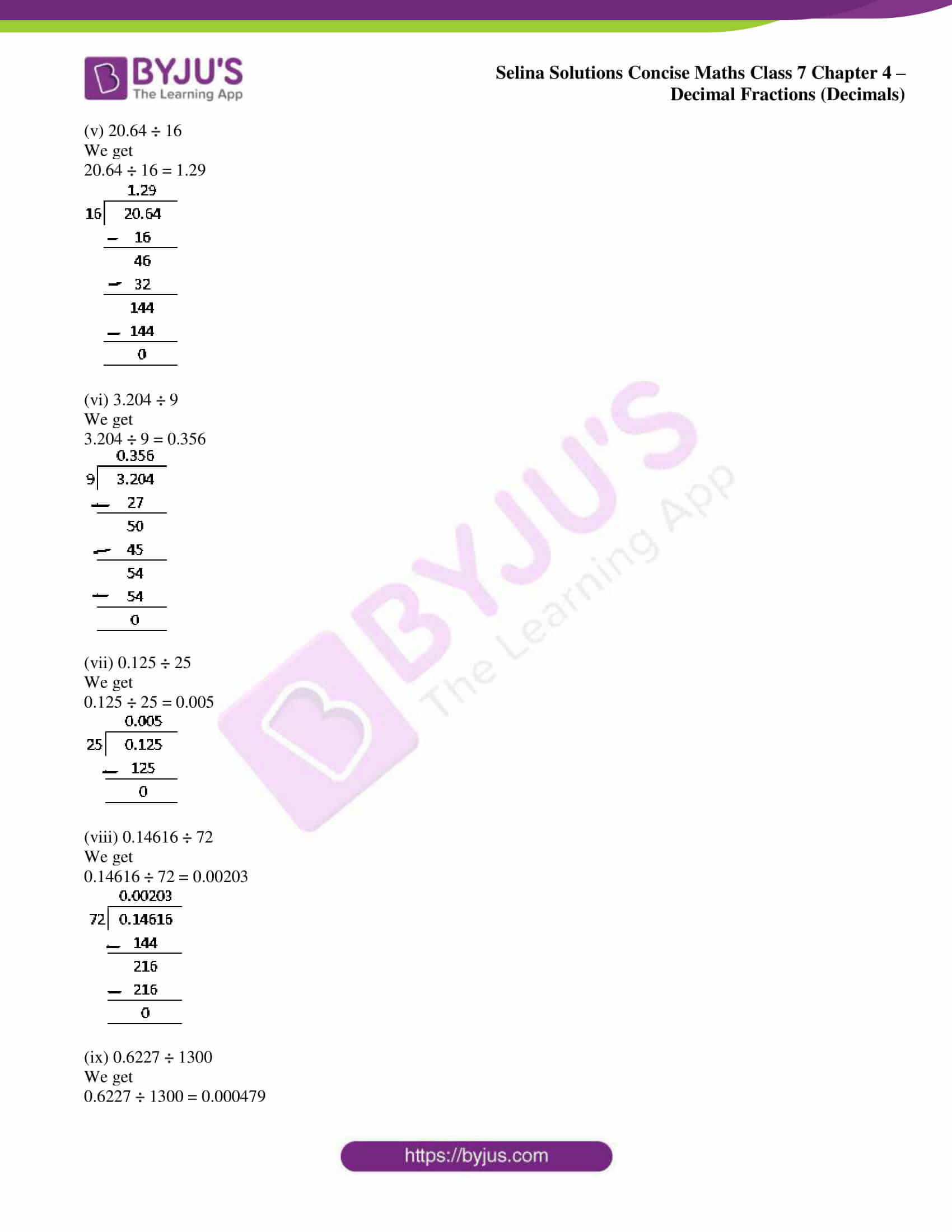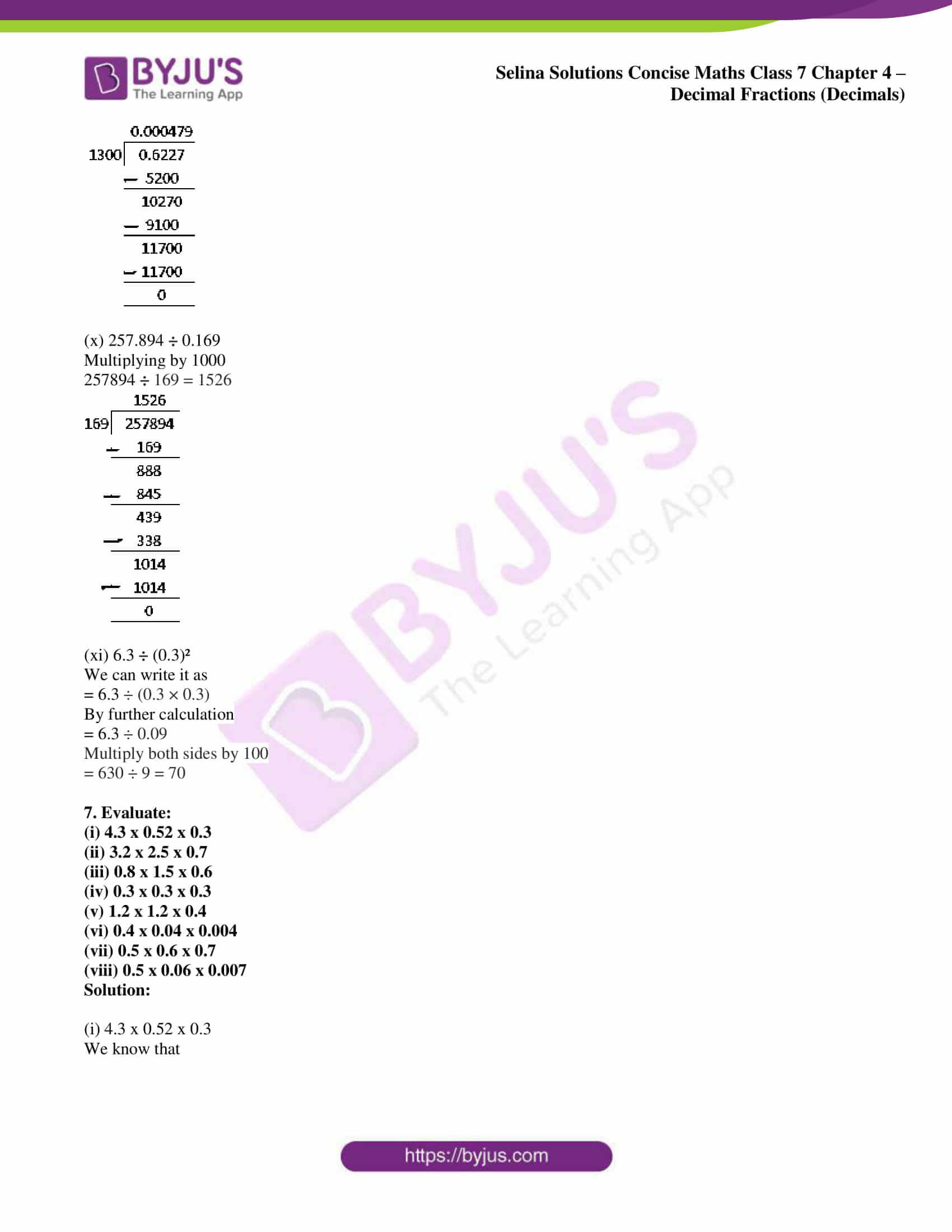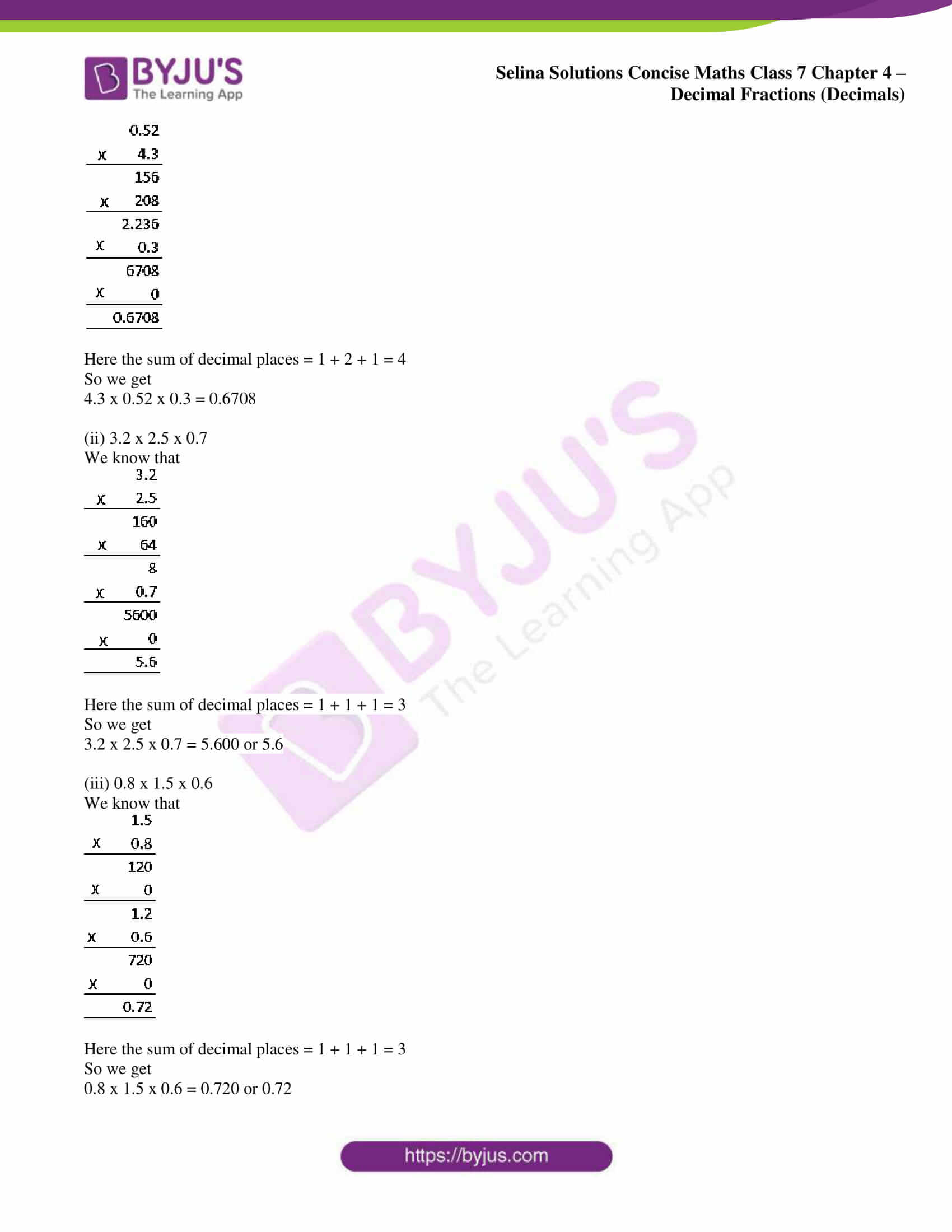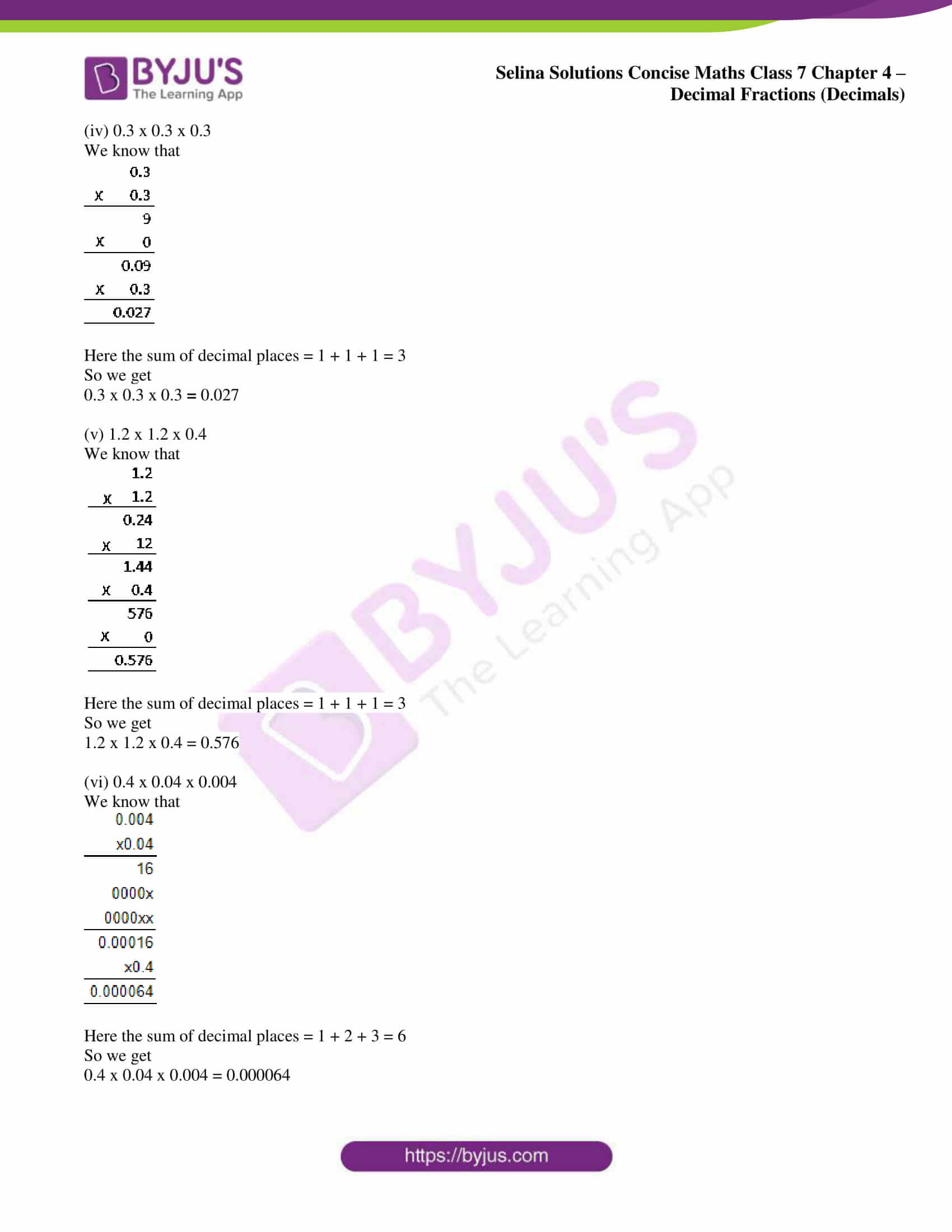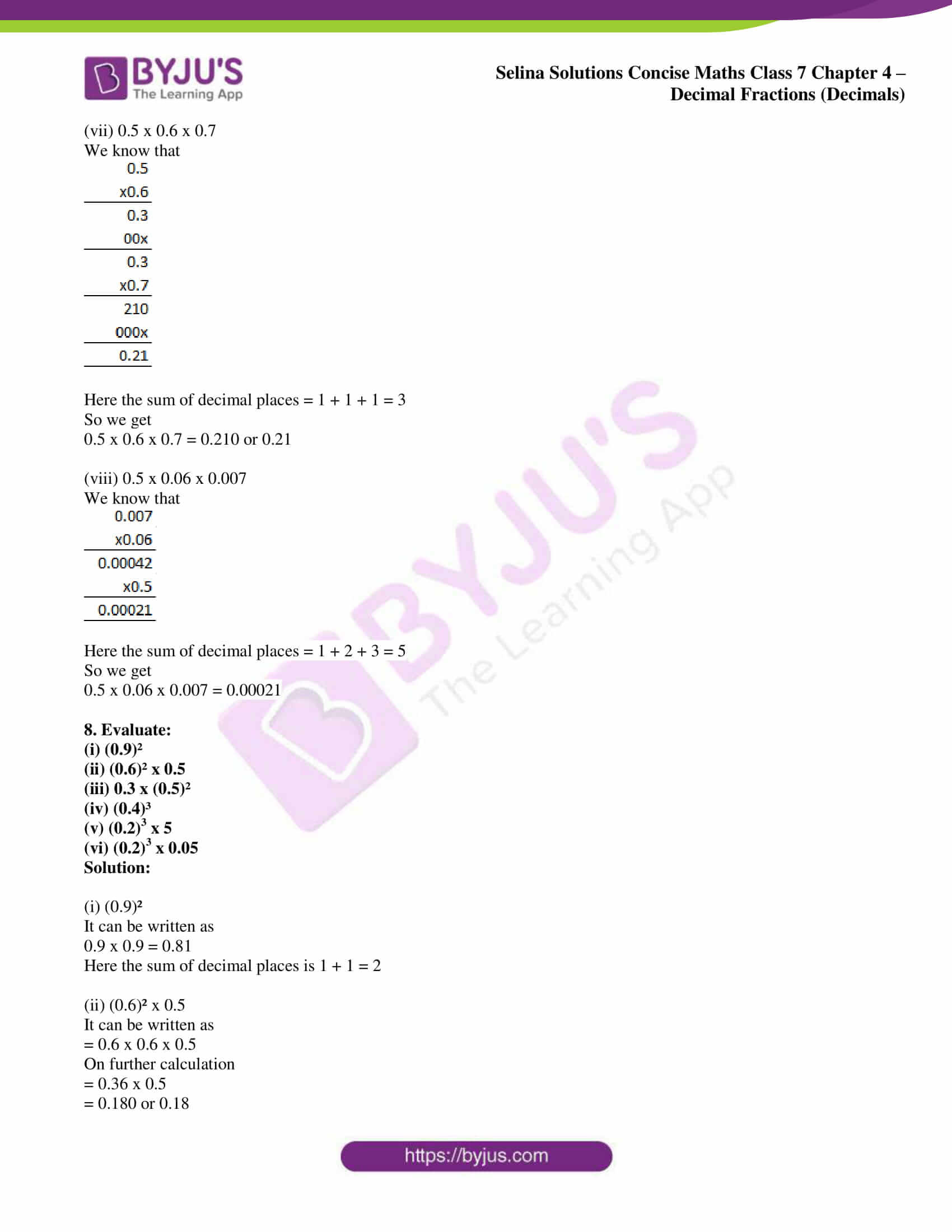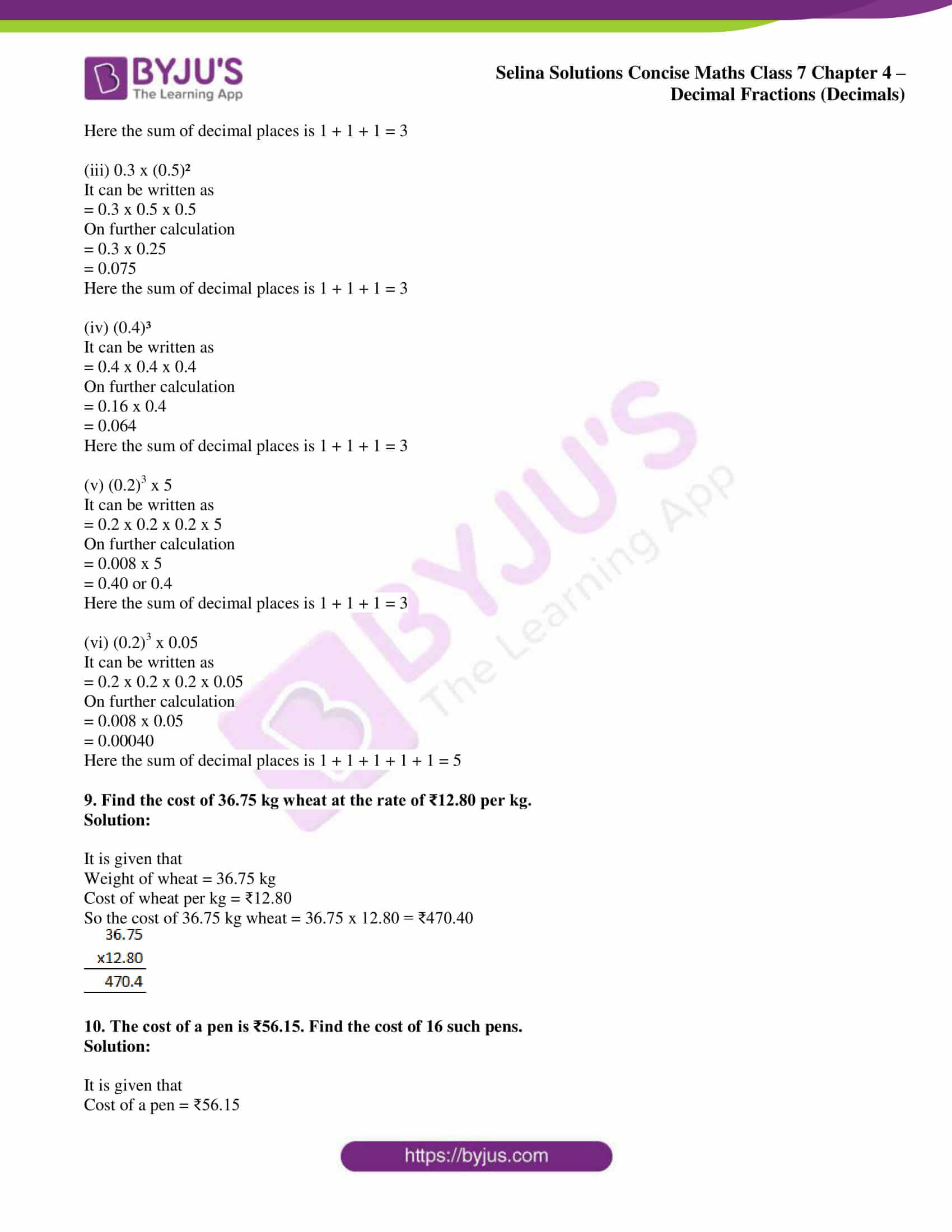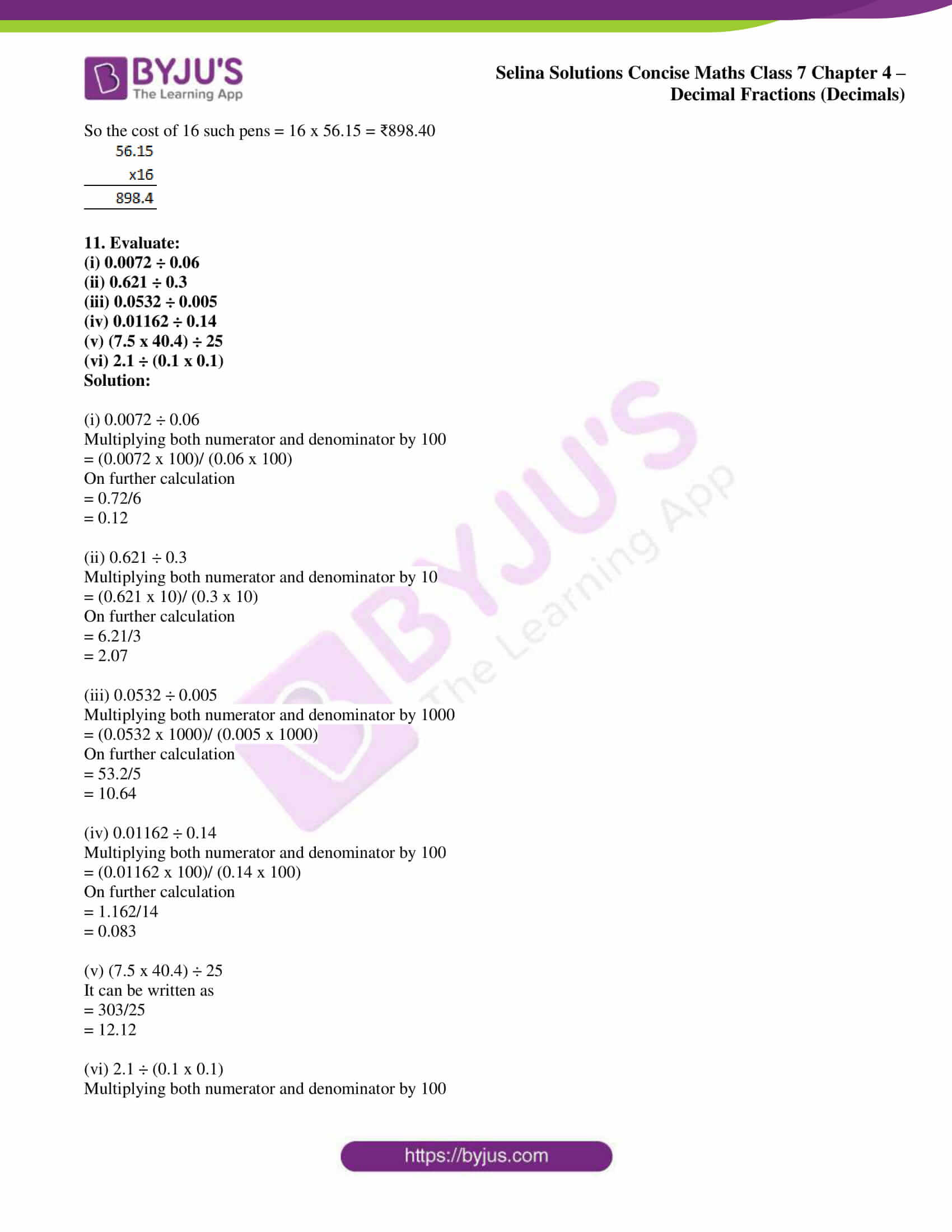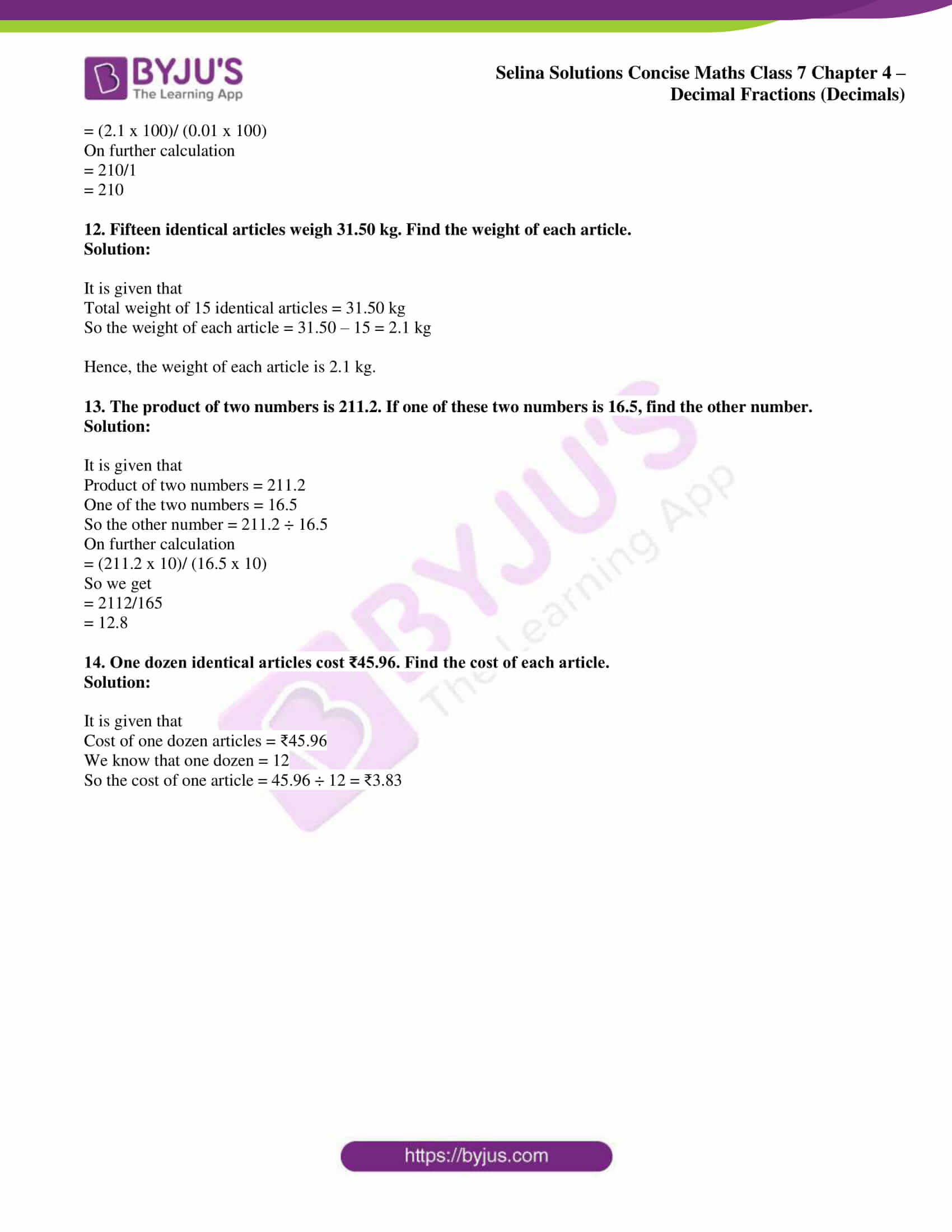### Access Selina Solutions Concise Maths Class 7 Chapter 4: Decimal Fractions (Decimals) Exercise 4C

1. Multiply:

(i) 0.87 by 10

(ii) 2.948 by 100

(iii) 6.4 by 1000

(iv) 5.8 by 4

(v) 16.32 by 28

(vi) 5. 037 by 8

(vi) 4.6 by 2.1

(viii) 0.568 by 6.4

Solution:

(i) 0.87 by 10
It can be written as

0.87 × 10 = 8.7

(ii) 2.948 by 100
It can be written as

2.948 × 100 = 294.8

(iii) 6.4 by 1000
It can be written as

6.4 × 1000 = 6400

(iv) 5.8 by 4
It can be written as

5.8 × 4 = 23.2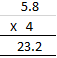(v) 16.32 by 28
It can be written as

16.32 × 28 = 456.96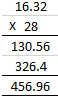(vi) 5.037 by 8
It can be written as

5.037 × 8 = 40.296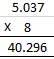(vi) 4.6 by 2.1
It can be written as

4.6 × 2.1 = 9.66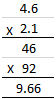(viii) 0.568 by 6.4

It can be written as

0.568 × 6.4 = 3.6352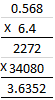2. Multiply each number by 10, 100, 1000:

(i) 0.5

(ii) 0.112

(iii) 4.8

(iv) 0.0359

(v) 16.27

(vi) 234.8

Solution:

(i) 0.5
It can be written as

0.5 × 10 = 5

0.5 × 100 = 50

0.5 × 1000 = 500

(ii) 0.112
It can be written as

0.112 × 10 = 1.12

0.112 × 100 = 11.2

0.112 × 1000 = 112

(iii) 4.8
It can be written as

4.8 × 10 = 48

4.8 × 100 = 480

4.8 × 1000 = 4800

(iv) 0.0359
It can be written as

0.0359 × 10 = 0.359

0.0359 × 100 = 3.59

0.0359 × 1000 = 35.9

(v) 16.27
It can be written as

16.27 × 10 = 162.7

16.27 × 100 = 1627

16.27 × 1000 = 16270

(vi) 234.8

It can be written as

234.8 × 10 = 2348

234.8 × 100 = 23480

234.8 × 1000 = 234800

3. Evaluate:

(i) 5.897 x 2.3

(ii) 0.894 x 87

(iii) 0.01 x 0.001

(iv) 0.84 x 2.2 x 4

(v) 4.75 x 0.08 x 3

(vi) 2.4 x 3.5 x 4.8

(vii) 0.8 x 1.2 x 0.25

(viii) 0.3 x 0.03 x 0.003

Solution:

(i) 5.897 x 2.3
We know that

5.897 x 2.3 = 13.5631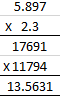(ii) 0.894 x 87
We know that

0.894 x 87 = 77.778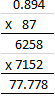(iii) 0.01 x 0.001
We know that

0.01 x 0.001 = 0.00001

(iv) 0.84 x 2.2 x 4
It can be written as

= 0.84 x 8.8

= 7.392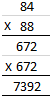(v) 4.75 x 0.08 x 3
It can be written as

= 4.75 x 0.24

= 1.1400

= 1.14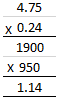(vi) 2.4 x 3.5 x 4.8
It can be written as

= 8.40 x 4.8

= 8.4 x 4.8

We get

= 40.32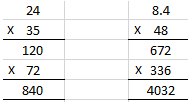(vii) 0.8 x 1.2 x 0.25
It can be written as

= 0.96 x 0.25
= 0.2400

= 0.24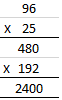(viii) 0.3 x 0.03 x 0.003

It can be written as

= 0.009 x 0.003

= 0.000027

4. Divide:

(i) 54.9 by 10

(ii) 7.8 by 100

(iii) 324.76 by 1000

(iv) 12.8 by 4

(v) 27.918 by 9

(vi) 4.672 by 8

(vii) 4.32 by 1.2

(viii) 7.644 by 1.4

(ix) 4.8432 by 0.08

Solution:

(i) 54.9 by 10
It can be written as

54.9 ÷ 10 = 5.49

(ii) 7.8 by 100
It can be written as

7.8 ÷ 100 = 0.078

(iii) 324.76 by 1000
It can be written as

324.76 ÷ 1000 = 0.32476

(iv) 12.8 by 4
It can be written as

12.8 ÷ 4 = 3.2

(v) 27.918 by 9
It can be written as

27.918 ÷ 9 = 3.102

(vi) 4.672 by 8

It can be written as

4.672 ÷ 8 = 0.584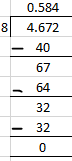(vii) 4.32 by 1.2
It can be written as

4.32 ÷ 1.2

Multiplying by 100

432 ÷ 120 = 3.6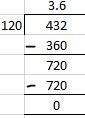(viii) 7.644 by 1.4
It can be written as

7.644 ÷ 1.4

Multiplying by 1000

7644 ÷ 1400 = 5.46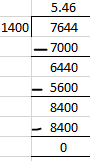(ix) 4.8432 by 0.08

It can be written as

4.8432 ÷ 0.08

So we get

48432 ÷ 800 = 60.54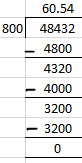5. Divide each of the given numbers by 10, 100, 1000 and 10000

(i) 2.1

(ii) 8.64

(iii) 5-01

(iv) 0.0906

(v) 0.125

(vi) 111.11

Solution:

(i) 2.1
It can be written as

2.1 ÷ 10 = 0.21

2.1 ÷ 100 = 0.021

2.1 ÷ 1000 = 0.0021

2.1 ÷ 10000 = 0.00021

(ii) 8.64
It can be written as

8.64 ÷ 10 = 0.864

8.64 ÷ 100 = 0.0864

8.64 ÷ 1000 = 0.00864

8.64 ÷ 10000 = 0.000864

(iii) 5.01
It can be written as

5.01 ÷ 10 = 0.501

5.01 ÷ 100 = 0.0501

5.01 ÷ 1000 = 0.00501

5.01 ÷ 10000 = 0.000501

(iv) 0.0906
It can be written as

0.0906 ÷ 10 = 0.00906

0.0906 ÷ 100 = 0.000906

0.0906 ÷ 1000 = 0.0000906

0.0906 ÷ 10000 = 0.00000906

(v) 0.125
It can be written as

0.125 ÷ 10 = 0.0125

0.125 ÷ 100 = 0.00125

0.125 ÷ 1000 = 0.000125

0.125 ÷ 10000 = 0.0000125

(vi) 111.11

It can be written as

111.11 ÷ 10 = 11.111

111.11 ÷ 100 = 1.1111

111.11 ÷ 1000 = 0.11111

111.11 ÷ 10000 = 0.011111

6. Evaluate :

(i) 9.75 ÷ 5

(ii) 4.4064 ÷ 4

(iii) 27.69 ÷ 30

(iv) 19.25 ÷ 25

(v) 20.64 ÷ 16

(vi) 3.204 ÷ 9

(vii) 0.125 ÷ 25

(viii) 0.14616 ÷ 72

(ix) 0.6227 ÷ 1300

(x) 257.894 ÷ 0.169

(xi) 6.3 ÷ (0.3)²

Solution:

(i) 9.75 ÷ 5
We get

9.75 ÷ 5 = 1.95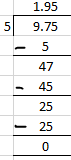(ii) 4.4064 ÷ 4
We get

4.4064 ÷ 4 = 1.016

(iii) 27.69 ÷ 30
We get

27.69 ÷ 30 = 0.923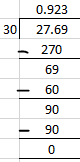(iv) 19.25 ÷ 25
We get

19.25 ÷ 25 = 0.77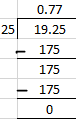(v) 20.64 ÷ 16
We get

20.64 ÷ 16 = 1.29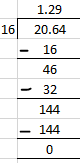(vi) 3.204 ÷ 9
We get

3.204 ÷ 9 = 0.356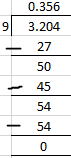(vii) 0.125 ÷ 25
We get

0.125 ÷ 25 = 0.005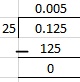(viii) 0.14616 ÷ 72
We get

0.14616 ÷ 72 = 0.00203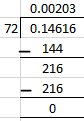(ix) 0.6227 ÷ 1300
We get

0.6227 ÷ 1300 = 0.000479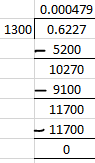(x) 257.894 ÷ 0.169
Multiplying by 1000

257894 ÷ 169 = 1526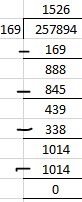(xi) 6.3 ÷ (0.3)²

We can write it as

= 6.3 ÷ (0.3 × 0.3)

By further calculation

= 6.3 ÷ 0.09

Multiply both sides by 100

= 630 ÷ 9 = 70

7. Evaluate:

(i) 4.3 x 0.52 x 0.3

(ii) 3.2 x 2.5 x 0.7

(iii) 0.8 x 1.5 x 0.6

(iv) 0.3 x 0.3 x 0.3

(v) 1.2 x 1.2 x 0.4

(vi) 0.4 x 0.04 x 0.004

(vii) 0.5 x 0.6 x 0.7

(viii) 0.5 x 0.06 x 0.007

Solution:

(i) 4.3 x 0.52 x 0.3
We know that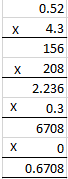Here the sum of decimal places = 1 + 2 + 1 = 4

So we get

4.3 x 0.52 x 0.3 = 0.6708

(ii) 3.2 x 2.5 x 0.7
We know that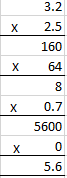Here the sum of decimal places = 1 + 1 + 1 = 3

So we get

3.2 x 2.5 x 0.7 = 5.600 or 5.6

(iii) 0.8 x 1.5 x 0.6
We know that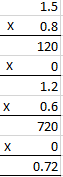Here the sum of decimal places = 1 + 1 + 1 = 3

So we get

0.8 x 1.5 x 0.6 = 0.720 or 0.72

(iv) 0.3 x 0.3 x 0.3
We know that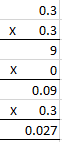Here the sum of decimal places = 1 + 1 + 1 = 3

So we get

0.3 x 0.3 x 0.3 = 0.027

(v) 1.2 x 1.2 x 0.4
We know that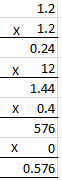Here the sum of decimal places = 1 + 1 + 1 = 3

So we get

1.2 x 1.2 x 0.4 = 0.576

(vi) 0.4 x 0.04 x 0.004
We know that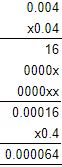Here the sum of decimal places = 1 + 2 + 3 = 6

So we get

0.4 x 0.04 x 0.004 = 0.000064

(vii) 0.5 x 0.6 x 0.7

We know that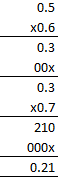Here the sum of decimal places = 1 + 1 + 1 = 3

So we get

0.5 x 0.6 x 0.7 = 0.210 or 0.21

(viii) 0.5 x 0.06 x 0.007

We know that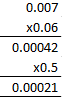Here the sum of decimal places = 1 + 2 + 3 = 5

So we get

0.5 x 0.06 x 0.007 = 0.00021

8. Evaluate:

(i) (0.9)²

(ii) (0.6)² x 0.5

(iii) 0.3 x (0.5)²

(iv) (0.4)³

(v) (0.2)3 x 5

(vi) (0.2)3 x 0.05

Solution:

(i) (0.9)²
It can be written as

0.9 x 0.9 = 0.81

Here the sum of decimal places is 1 + 1 = 2

(ii) (0.6)² x 0.5
It can be written as

= 0.6 x 0.6 x 0.5

On further calculation

= 0.36 x 0.5

= 0.180 or 0.18

Here the sum of decimal places is 1 + 1 + 1 = 3

(iii) 0.3 x (0.5)²
It can be written as

= 0.3 x 0.5 x 0.5

On further calculation

= 0.3 x 0.25

= 0.075

Here the sum of decimal places is 1 + 1 + 1 = 3

(iv) (0.4)³
It can be written as

= 0.4 x 0.4 x 0.4

On further calculation

= 0.16 x 0.4

= 0.064

Here the sum of decimal places is 1 + 1 + 1 = 3

(v) (0.2)3 x 5
It can be written as

= 0.2 x 0.2 x 0.2 x 5

On further calculation

= 0.008 x 5

= 0.40 or 0.4

Here the sum of decimal places is 1 + 1 + 1 = 3

(vi) (0.2)3 x 0.05

It can be written as

= 0.2 x 0.2 x 0.2 x 0.05

On further calculation

= 0.008 x 0.05

= 0.00040

Here the sum of decimal places is 1 + 1 + 1 + 1 + 1 = 5

9. Find the cost of 36.75 kg wheat at the rate of ₹12.80 per kg.

Solution:

It is given that

Weight of wheat = 36.75 kg

Cost of wheat per kg = ₹12.80

So the cost of 36.75 kg wheat = 36.75 x 12.80 = ₹470.40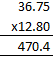10. The cost of a pen is ₹56.15. Find the cost of 16 such pens.

Solution:

It is given that

Cost of a pen = ₹56.15

So the cost of 16 such pens = 16 x 56.15 = ₹898.40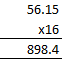11. Evaluate:

(i) 0.0072 ÷ 0.06

(ii) 0.621 ÷ 0.3

(iii) 0.0532 ÷ 0.005

(iv) 0.01162 ÷ 0.14

(v) (7.5 x 40.4) ÷ 25

(vi) 2.1 ÷ (0.1 x 0.1)

Solution:

(i) 0.0072 ÷ 0.06
Multiplying both numerator and denominator by 100

= (0.0072 x 100)/ (0.06 x 100)

On further calculation

= 0.72/6

= 0.12

(ii) 0.621 ÷ 0.3
Multiplying both numerator and denominator by 10

= (0.621 x 10)/ (0.3 x 10)

On further calculation

= 6.21/3

= 2.07

(iii) 0.0532 ÷ 0.005
Multiplying both numerator and denominator by 1000

= (0.0532 x 1000)/ (0.005 x 1000)

On further calculation

= 53.2/5

= 10.64

(iv) 0.01162 ÷ 0.14
Multiplying both numerator and denominator by 100

= (0.01162 x 100)/ (0.14 x 100)

On further calculation

= 1.162/14

= 0.083

(v) (7.5 x 40.4) ÷ 25
It can be written as

= 303/25

= 12.12

(vi) 2.1 ÷ (0.1 x 0.1)

Multiplying both numerator and denominator by 100

= (2.1 x 100)/ (0.01 x 100)

On further calculation

= 210/1

= 210

12. Fifteen identical articles weigh 31.50 kg. Find the weight of each article.

Solution:

It is given that

Total weight of 15 identical articles = 31.50 kg

So the weight of each article = 31.50 – 15 = 2.1 kg

Hence, the weight of each article is 2.1 kg.

13. The product of two numbers is 211.2. If one of these two numbers is 16.5, find the other number.

Solution:

It is given that

Product of two numbers = 211.2

One of the two numbers = 16.5

So the other number = 211.2 ÷ 16.5

On further calculation

= (211.2 x 10)/ (16.5 x 10)

So we get

= 2112/165

= 12.8

14. One dozen identical articles cost ₹45.96. Find the cost of each article.

Solution:

It is given that

Cost of one dozen articles = ₹45.96

We know that one dozen = 12

So the cost of one article = 45.96 ÷ 12 = ₹3.83